SQL functions are built into Oracle Database and are available for use in various appropriate SQL statements. Do not confuse SQL functions with user-defined functions written in PL/SQL.

If you call a SQL function with an argument of a datatype other than the datatype expected by the SQL function, then Oracle attempts to convert the argument to the expected datatype before performing the SQL function.

Note:

The combined values of the `NLS_COMP` and `NLS_SORT` settings determine the rules by which characters are sorted and compared. If `NLS_COMP` is set to `LINGUISTIC` for your database, then all entities in this chapter will be interpreted according to the rules specified by the `NLS_SORT` parameter. If `NLS_COMP` is not set to `LINGUISTIC`, then the functions are interpreted without regard to the `NLS_SORT` setting. `NLS_SORT` can be explicitly set. If it is not set explicitly, it is derived from `NLS_LANGUAGE`. Please refer to Oracle Database Globalization Support Guide for more information on these settings.

In the syntax diagrams for SQL functions, arguments are indicated by their datatypes. When the parameter `function` appears in SQL syntax, replace it with one of the functions described in this section. Functions are grouped by the datatypes of their arguments and their return values.

Note:

When you apply SQL functions to LOB columns, Oracle Database creates temporary LOBs during SQL and PL/SQL processing. You should ensure that temporary tablespace quota is sufficient for storing these temporary LOBs for your application.

The syntax showing the categories of functions follows:

function::=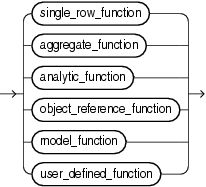Description of the illustration function.gif

single_row_function::=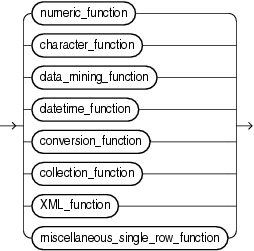Description of the illustration single_row_function.gif

The sections that follow list the built-in SQL functions in each of the groups illustrated in the preceding diagrams except user-defined functions. All of the built-in SQL functions are then described in alphabetical order.

## Single-Row Functions

Single-row functions return a single result row for every row of a queried table or view. These functions can appear in select lists, `WHERE` clauses, `START` `WITH` and `CONNECT` `BY` clauses, and `HAVING` clauses.

### Numeric Functions

Numeric functions accept numeric input and return numeric values. Most numeric functions that return `NUMBER` values that are accurate to 38 decimal digits. The transcendental functions `COS`, `COSH`, `EXP`, `LN`, `LOG`, `SIN`, `SINH`, `SQRT`, `TAN`, and `TANH` are accurate to 36 decimal digits. The transcendental functions `ACOS`, `ASIN`, `ATAN`, and `ATAN2` are accurate to 30 decimal digits. The numeric functions are:

ABS
ACOS
ASIN
ATAN
ATAN2
BITAND
CEIL
COS
COSH
EXP
FLOOR
LN
LOG
MOD
NANVL
POWER
REMAINDER
ROUND (number)
SIGN
SIN
SINH
SQRT
TAN
TANH
TRUNC (number)
WIDTH_BUCKET

### Character Functions Returning Character Values

Character functions that return character values return values of the following datatypes unless otherwise documented:

• If the input argument is `CHAR` or `VARCHAR2`, then the value returned is `VARCHAR2`.

• If the input argument is `NCHAR` or `NVARCHAR2`, then the value returned is `NVARCHAR2`.

The length of the value returned by the function is limited by the maximum length of the datatype returned.

• For functions that return `CHAR` or `VARCHAR2`, if the length of the return value exceeds the limit, then Oracle Database truncates it and returns the result without an error message.

• For functions that return `CLOB` values, if the length of the return values exceeds the limit, then Oracle raises an error and returns no data.

The character functions that return character values are:

CHR
CONCAT
INITCAP
LOWER
LTRIM
NLS_INITCAP
NLS_LOWER
NLSSORT
NLS_UPPER
REGEXP_REPLACE
REGEXP_SUBSTR
REPLACE
RTRIM
SOUNDEX
SUBSTR
TRANSLATE
TREAT
TRIM
UPPER

### NLS Character Functions

The NLS character functions return information about the character set. The NLS character functions are:

NLS_CHARSET_DECL_LEN
NLS_CHARSET_ID
NLS_CHARSET_NAME

### Character Functions Returning Number Values

Character functions that return number values can take as their argument any character datatype.

The character functions that return number values are:

ASCII
INSTR
LENGTH
REGEXP_INSTR

### Datetime Functions

Datetime functions operate on date (`DATE`), timestamp (`TIMESTAMP`, `TIMESTAMP` `WITH` `TIME` `ZONE`, and `TIMESTAMP` `WITH` `LOCAL` `TIME` `ZONE`), and interval (`INTERVAL` `DAY` `TO` `SECOND`, `INTERVAL` `YEAR` `TO` `MONTH`) values.

Some of the datetime functions were designed for the Oracle `DATE` datatype (`ADD_MONTHS`, `CURRENT_DATE`, `LAST_DAY`, `NEW_TIME`, and `NEXT_DAY`). If you provide a timestamp value as their argument, then Oracle Database internally converts the input type to a `DATE` value and returns a `DATE` value. The exceptions are the `MONTHS_BETWEEN` function, which returns a number, and the `ROUND` and `TRUNC` functions, which do not accept timestamp or interval values at all.

The remaining datetime functions were designed to accept any of the three types of data (date, timestamp, and interval) and to return a value of one of these types.

All of the datetime functions that return current system datetime information, such as `SYSDATE`, `SYSTIMESTAMP`, `CURRENT_TIMESTAMP`, and so forth, are evaluated once for each SQL statement, regardless how many times they are referenced in that statement.

The datetime functions are:

CURRENT_DATE
CURRENT_TIMESTAMP
DBTIMEZONE
EXTRACT (datetime)
FROM_TZ
LAST_DAY
LOCALTIMESTAMP
MONTHS_BETWEEN
NEW_TIME
NEXT_DAY
NUMTODSINTERVAL
NUMTOYMINTERVAL
ROUND (date)
SESSIONTIMEZONE
SYS_EXTRACT_UTC
SYSDATE
SYSTIMESTAMP
TO_CHAR (datetime)
TO_TIMESTAMP
TO_TIMESTAMP_TZ
TO_DSINTERVAL
TO_YMINTERVAL
TRUNC (date)
TZ_OFFSET

### General Comparison Functions

The general comparison functions determine the greatest and or least value from a set of values. The general comparison functions are:

GREATEST
LEAST

### Conversion Functions

Conversion functions convert a value from one datatype to another. Generally, the form of the function names follows the convention `datatype` `TO` `datatype`. The first datatype is the input datatype. The second datatype is the output datatype. The SQL conversion functions are:

ASCIISTR
BIN_TO_NUM
CAST
CHARTOROWID
COMPOSE
CONVERT
DECOMPOSE
HEXTORAW
NUMTODSINTERVAL
NUMTOYMINTERVAL
RAWTOHEX
RAWTONHEX
ROWIDTOCHAR
ROWIDTONCHAR
SCN_TO_TIMESTAMP
TIMESTAMP_TO_SCN
TO_BINARY_DOUBLE
TO_BINARY_FLOAT
TO_CHAR (character)
TO_CHAR (datetime)
TO_CHAR (number)
TO_CLOB
TO_DATE
TO_DSINTERVAL
TO_LOB
TO_MULTI_BYTE
TO_NCHAR (character)
TO_NCHAR (datetime)
TO_NCHAR (number)
TO_NCLOB
TO_NUMBER
TO_DSINTERVAL
TO_SINGLE_BYTE
TO_TIMESTAMP
TO_TIMESTAMP_TZ
TO_YMINTERVAL
TO_YMINTERVAL
TRANSLATE ... USING
UNISTR

### Large Object Functions

The large object functions operate on LOBs. The large object functions are:

BFILENAME
EMPTY_BLOB, EMPTY_CLOB

### Collection Functions

The collection functions operate on nested tables and varrays. The SQL collection functions are:

CARDINALITY
COLLECT
POWERMULTISET
POWERMULTISET_BY_CARDINALITY
SET

### Hierarchical Function

The hierarchical function applies hierarchical path information to a result set.

SYS_CONNECT_BY_PATH

### Data Mining Functions

The data mining functions operate on models that have been built using the `DBMS_DATA_MINING` package or the Oracle Data Mining Java API. The SQL data mining functions are:

CLUSTER_ID
CLUSTER_PROBABILITY
CLUSTER_SET
FEATURE_ID
FEATURE_SET
FEATURE_VALUE
PREDICTION
PREDICTION_BOUNDS
PREDICTION_COST
PREDICTION_DETAILS
PREDICTION_PROBABILITY
PREDICTION_SET

### XML Functions

The XML functions operate on or return XML documents or fragments. For more information about selecting and querying XML data using these functions, including information on formatting output, refer to Oracle XML DB Developer's Guide. The SQL XML functions are:

APPENDCHILDXML
DELETEXML
DEPTH
EXTRACT (XML)
EXISTSNODE
EXTRACTVALUE
INSERTCHILDXML
INSERTXMLBEFORE
PATH
SYS_DBURIGEN
SYS_XMLAGG
SYS_XMLGEN
UPDATEXML
XMLAGG
XMLCAST
XMLCDATA
XMLCOLATTVAL
XMLCOMMENT
XMLCONCAT
XMLDIFF
XMLELEMENT
XMLEXISTS
XMLFOREST
XMLPARSE
XMLPATCH
XMLPI
XMLQUERY
XMLROOT
XMLSEQUENCE
XMLSERIALIZE
XMLTABLE
XMLTRANSFORM

### Encoding and Decoding Functions

The encoding and decoding functions let you inspect and decode data in the database.

DECODE
DUMP
ORA_HASH
VSIZE

### NULL-Related Functions

The `NULL`-related functions facilitate null handling. The `NULL`-related functions are:

COALESCE
LNNVL
NULLIF
NVL
NVL2

### Environment and Identifier Functions

The environment and identifier functions provide information about the instance and session. These functions are:

SYS_CONTEXT
SYS_GUID
SYS_TYPEID
UID
USER
USERENV

## Aggregate Functions

Aggregate functions return a single result row based on groups of rows, rather than on single rows. Aggregate functions can appear in select lists and in `ORDER` `BY` and `HAVING` clauses. They are commonly used with the `GROUP` `BY` clause in a `SELECT` statement, where Oracle Database divides the rows of a queried table or view into groups. In a query containing a `GROUP` `BY` clause, the elements of the select list can be aggregate functions, `GROUP` `BY` expressions, constants, or expressions involving one of these. Oracle applies the aggregate functions to each group of rows and returns a single result row for each group.

If you omit the `GROUP` `BY` clause, then Oracle applies aggregate functions in the select list to all the rows in the queried table or view. You use aggregate functions in the `HAVING` clause to eliminate groups from the output based on the results of the aggregate functions, rather than on the values of the individual rows of the queried table or view.

"Using the GROUP BY Clause: Examples" and the "HAVING Clause" for more information on the `GROUP` `BY` clause and `HAVING` clauses in queries and subqueries

Many (but not all) aggregate functions that take a single argument accept these clauses:

• `DISTINCT` causes an aggregate function to consider only distinct values of the argument expression.

• `ALL` causes an aggregate function to consider all values, including all duplicates.

For example, the `DISTINCT` average of 1, 1, 1, and 3 is 2. The `ALL` average is 1.5. If you specify neither, then the default is `ALL`.

Some aggregate functions allow the `windowing_clause`, which is part of the syntax of analytic functions. Refer to windowing_clause for information about this clause. In the listing of aggregate functions at the end of this section, the functions that allow the `windowing_clause` are followed by an asterisk (*)

All aggregate functions except `COUNT`(*) and `GROUPING` ignore nulls. You can use the `NVL` function in the argument to an aggregate function to substitute a value for a null. `COUNT` never returns null, but returns either a number or zero. For all the remaining aggregate functions, if the data set contains no rows, or contains only rows with nulls as arguments to the aggregate function, then the function returns null.

The aggregate functions `MIN`, `MAX`, `SUM`, `AVG`, `COUNT`, `VARIANCE`, and `STDDEV`, when followed by the `KEEP` keyword, can be used in conjunction with the `FIRST` or `LAST` function to operate on a set of values from a set of rows that rank as the `FIRST` or `LAST` with respect to a given sorting specification. Refer to FIRST for more information.

You can nest aggregate functions. For example, the following example calculates the average of the maximum salaries of all the departments in the sample schema `hr`:

```SELECT AVG(MAX(salary)) FROM employees GROUP BY department_id;

AVG(MAX(SALARY))
----------------
10925
```

This calculation evaluates the inner aggregate (`MAX`(`salary`)) for each group defined by the `GROUP` `BY` clause (`department_id`), and aggregates the results again.

The aggregate functions are:

AVG
COLLECT
CORR
CORR_*
COUNT
COVAR_POP
COVAR_SAMP
CUME_DIST
DENSE_RANK
FIRST
GROUP_ID
GROUPING
GROUPING_ID
LAST
MAX
MEDIAN
MIN
PERCENTILE_CONT
PERCENTILE_DISC
PERCENT_RANK
RANK
REGR_ (Linear Regression) Functions
STATS_BINOMIAL_TEST
STATS_CROSSTAB
STATS_F_TEST
STATS_KS_TEST
STATS_MODE
STATS_MW_TEST
STATS_ONE_WAY_ANOVA
STATS_T_TEST_*
STATS_WSR_TEST
STDDEV
STDDEV_POP
STDDEV_SAMP
SUM
VAR_POP
VAR_SAMP
VARIANCE

## Analytic Functions

Analytic functions compute an aggregate value based on a group of rows. They differ from aggregate functions in that they return multiple rows for each group. The group of rows is called a window and is defined by the `analytic_clause`. For each row, a sliding window of rows is defined. The window determines the range of rows used to perform the calculations for the current row. Window sizes can be based on either a physical number of rows or a logical interval such as time.

Analytic functions are the last set of operations performed in a query except for the final `ORDER` `BY` clause. All joins and all `WHERE`, `GROUP` `BY`, and `HAVING` clauses are completed before the analytic functions are processed. Therefore, analytic functions can appear only in the select list or `ORDER` `BY` clause.

Analytic functions are commonly used to compute cumulative, moving, centered, and reporting aggregates.

analytic_function::=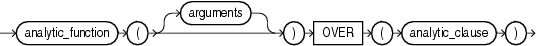Description of the illustration analytic_function.gif

analytic_clause::=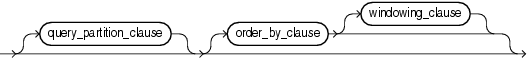Description of the illustration analytic_clause.gif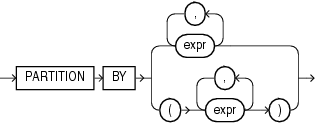Description of the illustration query_partition_clause.gif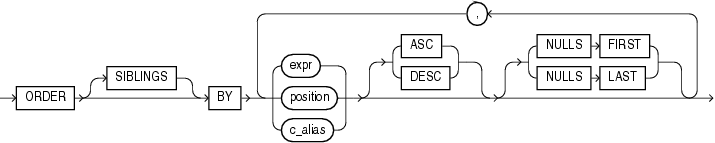Description of the illustration order_by_clause.gif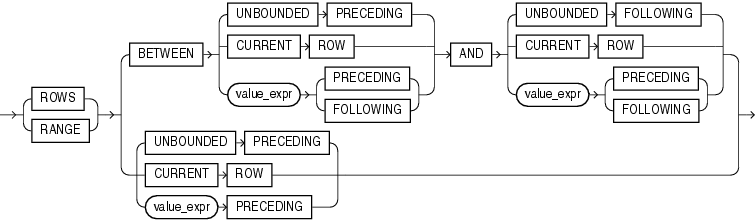Description of the illustration windowing_clause.gif

The semantics of this syntax are discussed in the sections that follow.

analytic_function

Specify the name of an analytic function (see the listing of analytic functions following this discussion of semantics).

arguments

Analytic functions take 0 to 3 arguments. The arguments can be any numeric datatype or any nonnumeric datatype that can be implicitly converted to a numeric datatype. Oracle determines the argument with the highest numeric precedence and implicitly converts the remaining arguments to that datatype. The return type is also that datatype, unless otherwise noted for an individual function.

"Numeric Precedence" for information on numeric precedence and Table 2-10, "Implicit Type Conversion Matrix" for more information on implicit conversion

analytic_clause

Use `OVER` `analytic_clause` to indicate that the function operates on a query result set. This clause is computed after the `FROM`, `WHERE`, `GROUP` `BY`, and `HAVING` clauses. You can specify analytic functions with this clause in the select list or `ORDER` `BY` clause. To filter the results of a query based on an analytic function, nest these functions within the parent query, and then filter the results of the nested subquery.

Notes on the analytic_clauseThe following notes apply to the `analytic_clause`:

• You cannot nest analytic functions by specifying any analytic function in any part of the `analytic_clause`. However, you can specify an analytic function in a subquery and compute another analytic function over it.

• You can specify `OVER` `analytic_clause` with user-defined analytic functions as well as built-in analytic functions. See CREATE FUNCTION.

query_partition_clause

Use the `PARTITION` `BY` clause to partition the query result set into groups based on one or more `value_expr`. If you omit this clause, then the function treats all rows of the query result set as a single group.

To use the `query_partition_clause` in an analytic function, use the upper branch of the syntax (without parentheses). To use this clause in a model query (in the `model_column_clauses`) or a partitioned outer join (in the `outer_join_clause`), use the lower branch of the syntax (with parentheses).

You can specify multiple analytic functions in the same query, each with the same or different `PARTITION` `BY` keys.

If the objects being queried have the parallel attribute, and if you specify an analytic function with the `query_partition_clause`, then the function computations are parallelized as well.

Valid values of `value_expr` are constants, columns, nonanalytic functions, function expressions, or expressions involving any of these.

order_by_clause

Use the `order_by_clause` to specify how data is ordered within a partition. For all analytic functions except `PERCENTILE_CONT` and `PERCENTILE_DISC` (which take only a single key), you can order the values in a partition on multiple keys, each defined by a `value_expr` and each qualified by an ordering sequence.

Within each function, you can specify multiple ordering expressions. Doing so is especially useful when using functions that rank values, because the second expression can resolve ties between identical values for the first expression.

Whenever the `order_by_clause` results in identical values for multiple rows, the function returns the same result for each of those rows. Refer to the analytic example for SUM for an illustration of this behavior.

Restrictions on the ORDER BY Clause The following restrictions apply to the `ORDER` `BY` clause:

• When used in an analytic function, the `order_by_clause` must take an expression (`expr`). The `SIBLINGS` keyword is not valid (it is relevant only in hierarchical queries). Position (`position`) and column aliases (`c_alias`) are also invalid. Otherwise this `order_by_clause` is the same as that used to order the overall query or subquery.

• An analytic function that uses the `RANGE` keyword can use multiple sort keys in its `ORDER` `BY` clause if it specifies either of these two windows:

• `RANGE` `BETWEEN` `UNBOUNDED` `PRECEDING` `AND` `CURRENT` `ROW`. The short form of this is `RANGE` `UNBOUNDED` `PRECEDING`.

• `RANGE` `BETWEEN` `CURRENT` `ROW` `AND` `UNBOUNDED` `FOLLOWING`.

Window boundaries other than these two can have only one sort key in the `ORDER` `BY` clause of the analytic function. This restriction does not apply to window boundaries specified by the `ROW` keyword.

ASC | DESC Specify the ordering sequence (ascending or descending). `ASC` is the default.

NULLS FIRST | NULLS LAST Specify whether returned rows containing nulls should appear first or last in the ordering sequence.

`NULLS` `LAST` is the default for ascending order, and `NULLS` `FIRST` is the default for descending order.

Analytic functions always operate on rows in the order specified in the `order_by_clause` of the function. However, the `order_by_clause` of the function does not guarantee the order of the result. Use the `order_by_clause` of the query to guarantee the final result ordering.

windowing_clause

Some analytic functions allow the `windowing_clause`. In the listing of analytic functions at the end of this section, the functions that allow the `windowing_clause` are followed by an asterisk (*).

ROWS | RANGE These keywords define for each row a window (a physical or logical set of rows) used for calculating the function result. The function is then applied to all the rows in the window. The window moves through the query result set or partition from top to bottom.

• `ROWS` specifies the window in physical units (rows).

• `RANGE` specifies the window as a logical offset.

You cannot specify this clause unless you have specified the `order_by_clause`. Some window boundaries defined by the `RANGE` clause let you specify only one expression in the `order_by_clause`. Refer to "Restrictions on the ORDER BY Clause".

The value returned by an analytic function with a logical offset is always deterministic. However, the value returned by an analytic function with a physical offset may produce nondeterministic results unless the ordering expression results in a unique ordering. You may have to specify multiple columns in the `order_by_clause` to achieve this unique ordering.

BETWEEN ... AND Use the `BETWEEN` ... `AND` clause to specify a start point and end point for the window. The first expression (before `AND`) defines the start point and the second expression (after `AND`) defines the end point.

If you omit `BETWEEN` and specify only one end point, then Oracle considers it the start point, and the end point defaults to the current row.

UNBOUNDED PRECEDING Specify `UNBOUNDED` `PRECEDING` to indicate that the window starts at the first row of the partition. This is the start point specification and cannot be used as an end point specification.

UNBOUNDED FOLLOWING Specify `UNBOUNDED` `FOLLOWING` to indicate that the window ends at the last row of the partition. This is the end point specification and cannot be used as a start point specification.

CURRENT ROW As a start point, `CURRENT` `ROW` specifies that the window begins at the current row or value (depending on whether you have specified `ROW` or `RANGE`, respectively). In this case the end point cannot be `value_expr` `PRECEDING`.

As an end point, `CURRENT` `ROW` specifies that the window ends at the current row or value (depending on whether you have specified `ROW` or `RANGE`, respectively). In this case the start point cannot be `value_expr` `FOLLOWING`.

value_expr PRECEDING or value_expr FOLLOWING For `RANGE` or `ROW`:

• If `value_expr` `FOLLOWING` is the start point, then the end point must be `value_expr` `FOLLOWING`.

• If `value_expr` `PRECEDING` is the end point, then the start point must be `value_expr` `PRECEDING`.

If you are defining a logical window defined by an interval of time in numeric format, then you may need to use conversion functions.

NUMTOYMINTERVAL and NUMTODSINTERVAL for information on converting numeric times into intervals

If you specified `ROWS`:

• `value_expr` is a physical offset. It must be a constant or expression and must evaluate to a positive numeric value.

• If `value_expr` is part of the start point, then it must evaluate to a row before the end point.

If you specified `RANGE`:

• `value_expr` is a logical offset. It must be a constant or expression that evaluates to a positive numeric value or an interval literal. Refer to "Literals" for information on interval literals.

• You can specify only one expression in the `order_by_clause`

• If `value_expr` evaluates to a numeric value, then the `ORDER` `BY` `expr` must be a numeric or `DATE` datatype.

• If `value_expr` evaluates to an interval value, then the `ORDER` `BY` `expr` must be a `DATE` datatype.

If you omit the `windowing_clause` entirely, then the default is `RANGE` `BETWEEN` `UNBOUNDED` `PRECEDING` `AND` `CURRENT` `ROW`.

Analytic functions are commonly used in data warehousing environments. In the list of analytic functions that follows, functions followed by an asterisk (*) allow the full syntax, including the `windowing_clause`.

AVG *
CORR *
COVAR_POP *
COVAR_SAMP *
COUNT *
CUME_DIST
DENSE_RANK
FIRST
FIRST_VALUE *
LAG
LAST
LAST_VALUE *
MAX *
MIN *
NTILE
PERCENT_RANK
PERCENTILE_CONT
PERCENTILE_DISC
RANK
RATIO_TO_REPORT
REGR_ (Linear Regression) Functions *
ROW_NUMBER
STDDEV *
STDDEV_POP *
STDDEV_SAMP *
SUM *
VAR_POP *
VAR_SAMP *
VARIANCE *

Oracle Database Data Warehousing Guide for more information on these functions and for scenarios illustrating their use

## Object Reference Functions

Object reference functions manipulate REF values, which are references to objects of specified object types. The object reference functions are:

DEREF
MAKE_REF
REF
REFTOHEX
VALUE

Oracle Database Object-Relational Developer's Guide for more information about `REF` datatypes

## Model Functions

Model functions can be used only in the `model_clause` of the `SELECT` statement. The model functions are:

CV
ITERATION_NUMBER
PRESENTNNV
PRESENTV
PREVIOUS

Press "Next" to go to the first SQL function.